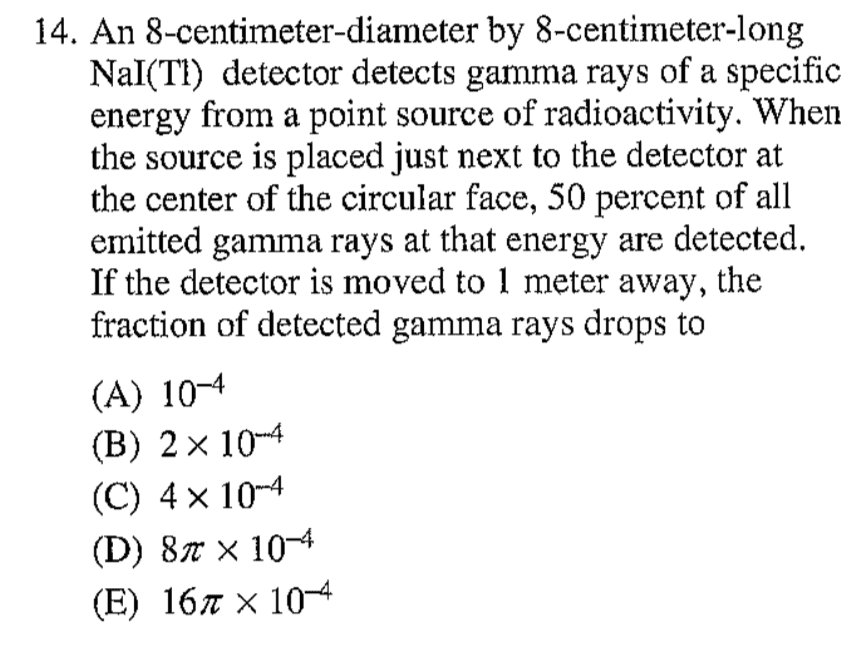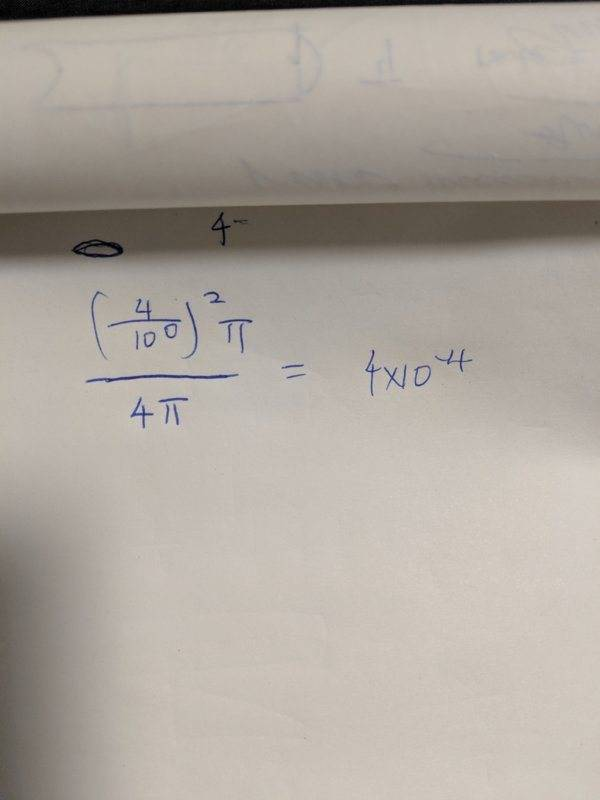# Can someone explain this GRE problem to me? (gamma ray detector)

• jdou86
But my answer comes nothing close to the choices below.In summary, the conversation involved trying to compute the area ratio between the end of a tube and a faraway distance, with the person's answer not matching the given choices. They were asked to provide their calculations and were given advice on how to properly solve the problem. However, the person expressed frustration with the required steps and eventually solved the problem with one line of math.f

#### jdou86

OP warned about not providing an attempt at a solution.How far did you get on this problem?

not far at all i was trying to compute the area raito btwn when it is at the end of the tube and far away. but my answer comes nothing close to the choices below

You'll have to provide an attempt at a solution to get help.

not far at all i was trying to compute the area raito btwn when it is at the end of the tube and far away. but my answer comes nothing close to the choices below
How are you using the bit about 50% being detected when the detector is placed against the face?

not far at all i was trying to compute the area raito btwn when it is at the end of the tube and far away. but my answer comes nothing close to the choices below
Please show these calculations, otherwise we can't tell what went wrong.

The solid angle is not proportional to the subtended angle. Rather, it relates to the area of the spherical cap of a sphere centred on the source, the cap spanning the aperture.
When the source is in the centre of the aperture, radius r, the end cap is a hemisphere of area ##2\pi r^2##. Dividing by the area of the whole sphere we get ##\frac{2\pi r^2}{4\pi r^2}=0.5##.
With the source at distance d from the centre of the aperture, the whole sphere has area##4\pi (r^2+d^2)##. The endcap's area has shrunk to not much more than ##\pi r^2##.
However, I am uncertain how the 8cm length fits in. If it is relevant then I don't understand how it can capture 50% initially.

I'm sorry, but if that's your attempt of a solution, you are not ready for graduate school.

You have expressions involving numbers, but no sense of what they are, where they come from, or where you are going with this.

If this is how you solve problems, when you get to Jackson or grad QM, you won't make it out the other end.

What you need to do is
1. Post a labeled drawing
2. Write down the relevant equation
3. Fill in the symbolic expressions in that equation and simplify
4. Now, and only now, plug in the numbers

•JD_PM and mfb
I'm sorry, but if that's your attempt of a solution, you are not ready for graduate school.

You have expressions involving numbers, but no sense of what they are, where they come from, or where you are going with this.

If this is how you solve problems, when you get to Jackson or grad QM, you won't make it out the other end.

What you need to do is
1. Post a labeled drawing
2. Write down the relevant equation
3. Fill in the symbolic expressions in that equation and simplify
4. Now, and only now, plug in the numbers
Often I just know the answer, labeling are left to the engineers

I'm sorry, but if that's your attempt of a solution, you are not ready for graduate school.

You have expressions involving numbers, but no sense of what they are, where they come from, or where you are going with this.

If this is how you solve problems, when you get to Jackson or grad QM, you won't make it out the other end.

What you need to do is
1. Post a labeled drawing
2. Write down the relevant equation
3. Fill in the symbolic expressions in that equation and simplify
4. Now, and only now, plug in the numbers
And this is physics GREthere is no time for all these steps you wantedI just solved the problem with two l
The solid angle is not proportional to the subtended angle. Rather, it relates to the area of the spherical cap of a sphere centred on the source, the cap spanning the aperture.
When the source is in the centre of the aperture, radius r, the end cap is a hemisphere of area ##2\pi r^2##. Dividing by the area of the whole sphere we get ##\frac{2\pi r^2}{4\pi r^2}=0.5##.
With the source at distance d from the centre of the aperture, the whole sphere has area##4\pi (r^2+d^2)##. The endcap's area has shrunk to not much more than ##\pi r^2##.
However, I am uncertain how the 8cm length fits in. If it is relevant then I don't understand how it can capture 50% initially.
Thanks to you I solved it with 1 line of math

I'm sorry, but if that's your attempt of a solution, you are not ready for graduate school.

You have expressions involving numbers, but no sense of what they are, where they come from, or where you are going with this.

If this is how you solve problems, when you get to Jackson or grad QM, you won't make it out the other end.

What you need to do is
1. Post a labeled drawing
2. Write down the relevant equation
3. Fill in the symbolic expressions in that equation and simplify
4. Now, and only now, plug in the numbers
And fyi your response is both rude and ignorant. I don't think landau did any of the labeling crap. But discovered phase transition.

Often I just know the answer, labeling are left to the engineers

And when you don't know what the answer is? You're not going to be able to ask us when you're taking the GRE.

Anyway, It doesn't really matter if you don't want to take our advice. This will fix itself sooner or later.

And when you don't know what the answer is? You're not going to be able to ask us when you're taking the GRE.

Anyway, It doesn't really matter if you don't want to take our advice. This will fix itself sooner or later.
Labeling was never the problem I think you are too arrogant to see that. Sure. So as your ego

fyi your response is both rude and ignorant

I think you are too arrogant to see that

This attitude just got you a warning and a closed thread. Please be civil to others when you come here asking for help with a problem.

Also, for future reference, you should not post equations or solutions as images. Please use the PF LaTeX feature to post those directly:

https://www.physicsforums.com/help/latexhelp/

•JD_PM